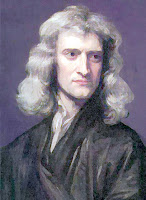## Wednesday, 1 August 2012

### CBSE Class 9 - Science - CH9 - Force and Laws of Motion (worksheet)Isaac Newton
Force and Laws of Motion
Worksheet
Fill in the blanks

1. The study of motion of a body under the action of a force is called ______.

2. (Impulse/Force/Pressure) ______ is a push or pull acting upon an object.

3. The natural tendency of objects to resist a change in their state of rest or of uniform motion is called ______.

4. When the resultant of all the forces acting on a body is zero, the forces are _______ (balanced/unbalanced).

5. When The resultant of all the forces acting on a body is not zero, the forces are _______ (balanced/unbalanced).

6.  Newton’s first law of motion states that a body at rest will remain at _____ (rest/motion) and a body in motion will remain in ________ (uniform/non-uniform) motion unless acted upon by an
_________ (balanced/unbalanced) force.

7. The ______  of an object is a measure of its inertia.

8. _________  (Nuclear/Gravitational/Muscular) Force is the weakest force

9. _______ (Nuclear/Gravitational/Muscular) force is the strongest force of all the basic forces in nature.

10.  An imaginary co-ordinate system which is either at rest or in uniform and where Newton’s laws are valid is called an _______ frame of reference.

11. (Centrifugal/Centripetal) ________  force is the apparent outward force that draws a rotating body away from the center of rotation.

12. The radially inward force towards the centre which continuously deflects a particle from its straight line path and makes it along a circular path is called _______ (centrifugal/centripetal) force.

13. The momentum of an object is the product of its _____ and _______ and has the same direction as that of the ________.

14. Newton’s second law of motion states that the rate of change of ______ of a body is directly proportional to the force and takes place in the same direction as the ______.

15. Action and reaction forces act on ________ (the same body/two different bodies)  but they are ______ (equal/unequal) in magnitude.

16. When the resultant force acting on a system is zero , the total momentum of the system  ______ (remains constant/ may vary). This is called ___________________ .

17. The velocity with which gun moves in the backward direction is known as the ______ velocity.

18. When the lift is stationary or moves with uniform velocity then acceleration is zero and
net force is ______ (zero/non-zero).

19. A change in momentum over a longer time requires ______ (less/more) force

1. dynamics
2. Force
3. inertia
4. balanced
5. unbalanced
6. rest, uniform, unbalanced
7. mass
8. Gravitational
9. Nuclear
10. inertial
11. Centrifugal
12. centripetal
13. mass, velocity, velocity
14. momentum, force
15. two different bodies, equal.
16. remains constant,  law of conservation of linear momentum
17. recoil
18. zero
19. less1.This comment has been removed by the author.

2.this site help us to know detail about this chapter and also help us in board exam

3.4.5.6.